Share

# Balbharati solutions for Maharashtra state board (SSC) Class 8 Mathematics chapter 2 - Parallel lines and transversals [Latest edition]

Textbook page

#### Chapters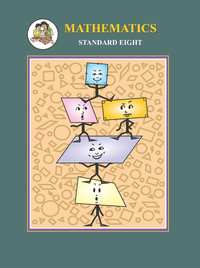## Chapter 2: Parallel lines and transversals

Practice Set 2.1Practice Set 2.2Practice Set 2.3

#### Balbharati solutions for Maharashtra state board (SSC) Class 8 Mathematics Chapter 2 Parallel lines and transversals Exercise Practice Set 2.1 [Page 8]

Practice Set 2.1 | Q 1 | Page 8

In the adjoining figure, each angle is shown by a letter. Fill in the boxes with the help of the figure.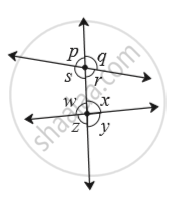Corresponding angles.

(1) ∠p and  ____
(2) ∠q and  ____
(3) ∠r and  ____
(4) ∠ s and _____ Interior alternate angles.
(5) ∠s and  _____
(6) ∠w and _____

Practice Set 2.1 | Q 1.1 | Page 8

Observe the angles shown in the figure and write the following pair of angle.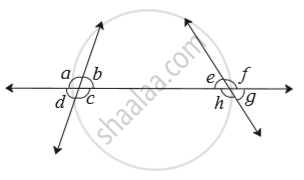Interior alternate angles

Practice Set 2.1 | Q 1.2 | Page 8

Observe the angles shown in the figure and write the following pair of angle.Corresponding angles

Practice Set 2.1 | Q 1.3 | Page 8

Observe the angles shown in the figure and write the following pair of angle.Interior angles

#### Balbharati solutions for Maharashtra state board (SSC) Class 8 Mathematics Chapter 2 Parallel lines and transversals Exercise Practice Set 2.2 [Pages 8 - 12]

Practice Set 2.2 | Q 1.1 | Page 11

Choose the correct alternative.

In the adjoining figure, if line m || line n and line p is a transversal then find x.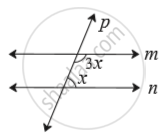• 135°

• 90°

• 45°

• 40°

Practice Set 2.2 | Q 1.2 | Page 8

Choose the correct alternative.

In the adjoining figure, if line a || line b and line l is a transversal then find x.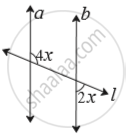• 90°

• 60°

• 45°

• 30°

Practice Set 2.2 | Q 2 | Page 11

In the adjoining figure line p || line q.  Line t and line s are transversals. Find measure of ∠x and ∠y using the measures of angles given in the figure.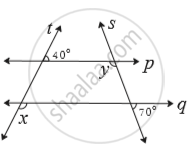Practice Set 2.2 | Q 3 | Page 12

In the adjoining figure. line p || line q.  line l || line m. Find measures of ∠a, ∠b, and ∠c, using the measures of given angles. Justify your answers.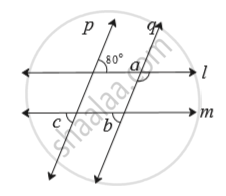Practice Set 2.2 | Q 4 | Page 12

In the adjoining figure, line a || line b.  line l is a transversal. Find the measures of ∠x, ∠y, ∠z using   the given information.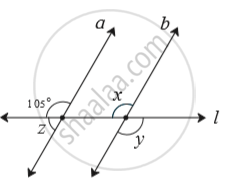Practice Set 2.2 | Q 5 | Page 12

In the adjoining figure, line p || line l || line q.  Find ∠x with the help of the measures given in the figure.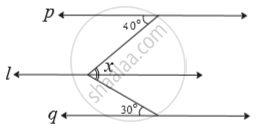#### Balbharati solutions for Maharashtra state board (SSC) Class 8 Mathematics Chapter 2 Parallel lines and transversals Exercise Practice Set 2.3 [Page 13]

Practice Set 2.3 | Q 1 | Page 13

Draw a line l. Take a point A outside the line. Through point A draw a line parallel to line l.

Practice Set 2.3 | Q 2 | Page 13

Draw a line l. Take a point T outside the line. Through point T draw a line parallel to line l.

Practice Set 2.3 | Q 3 | Page 13

Draw a line m. Draw a line n which is parallel to line m at a distance of 4 cm from it.

## Chapter 2: Parallel lines and transversals

Practice Set 2.1Practice Set 2.2Practice Set 2.3## Balbharati solutions for Maharashtra state board (SSC) Class 8 Mathematics chapter 2 - Parallel lines and transversals

Balbharati solutions for Maharashtra state board (SSC) Class 8 Mathematics chapter 2 (Parallel lines and transversals) include all questions with solution and detail explanation. This will clear students doubts about any question and improve application skills while preparing for board exams. The detailed, step-by-step solutions will help you understand the concepts better and clear your confusions, if any. Shaalaa.com has the Maharashtra State Board Maharashtra state board (SSC) Class 8 Mathematics solutions in a manner that help students grasp basic concepts better and faster.

Further, we at Shaalaa.com provide such solutions so that students can prepare for written exams. Balbharati textbook solutions can be a core help for self-study and acts as a perfect self-help guidance for students.

Concepts covered in Maharashtra state board (SSC) Class 8 Mathematics chapter 2 Parallel lines and transversals are Transversal, Properties of Angles Formed by Two Parallel Lines and a Transversal, To Draw a Line Parallel to the Given Line.

Using Balbharati Class 8 solutions Parallel lines and transversals exercise by students are an easy way to prepare for the exams, as they involve solutions arranged chapter-wise also page wise. The questions involved in Balbharati Solutions are important questions that can be asked in the final exam. Maximum students of Maharashtra State Board Class 8 prefer Balbharati Textbook Solutions to score more in exam.

Get the free view of chapter 2 Parallel lines and transversals Class 8 extra questions for Maharashtra state board (SSC) Class 8 Mathematics and can use Shaalaa.com to keep it handy for your exam preparation